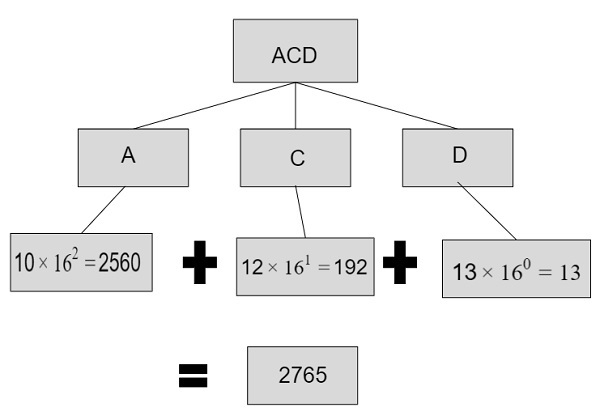# C++ program for hexadecimal to decimal

C++Server Side ProgrammingProgramming

Given with a hexadecimal number as an input, the task is to convert the given hexadecimal number into a decimal number.

Hexadecimal number in computers is represented with base 16 and decimal number is represented with base 10 and represented with values 0 - 9 whereas hexadecimal number have digits starting from 0 – 15 in which 10 is represented as A, 11 as B, 12 as C, 13 as D, 14 as E and 15 as F.

To convert a hexadecimal number into a decimal number follow these steps −

• We will extract digits starting from right to left through a remainder and then multiply it with the power starting from 0 and will be increased by 1 till the (number of digits) – 1
• Since we need to do conversions from hexdecimal to binary the base of power will be 16 as octal have base 16.
• Multiply the digits of given inputs with base and power and store the results
• Add all the multiplied values to obtain the final result which will be a decimal number.

Given below is the pictorial representation of converting a hexadecimal number into a decimal number.## Example

Input-: ACD
A(10) will be converted to a decimal number by -: 10 X 16^2 = 2560
C(12) will be converted to a decimal number by -: 12 X 16^1 = 192
D(13) will be converted to a decimal number by -: 13 X 16^0 = 13
Output-: total = 13 + 192 + 2560 = 2765

## Algorithm

Start
Step 1-> declare function to convert hexadecimal to decimal
int convert(char num[])
Set int len = strlen(num)
Set int base = 1
Set int temp = 0
Loop For int i=len-1 and i>=0 and i—
IF (num[i]>='0' && num[i]<='9')
Set temp += (num[i] - 48)*base
Set base = base * 16
End
Else If (num[i]>='A' && num[i]<='F')
Set temp += (num[i] - 55)*base
Set base = base*16
End
return temp
step 2-> In main()
declare char num[] = "3F456A"
Call convert(num)
Stop

## Example

Live Demo

#include<iostream>
#include<string.h>
using namespace std;
int convert(char num[]) {
int len = strlen(num);
int base = 1;
int temp = 0;
for (int i=len-1; i>=0; i--) {
if (num[i]>='0' && num[i]<='9') {
temp += (num[i] - 48)*base;
base = base * 16;
}
else if (num[i]>='A' && num[i]<='F') {
temp += (num[i] - 55)*base;
base = base*16;
}
}
return temp;
}
int main() {
char num[] = "3F456A";
cout<<num<<" after converting to deciaml becomes : "<<convert(num)<<endl;
return 0;
}

## Output

IF WE RUN THE ABOVE CODE IT WILL GENERATE FOLLOWING OUTPUT

3F456A after converting to deciaml becomes : 4146538